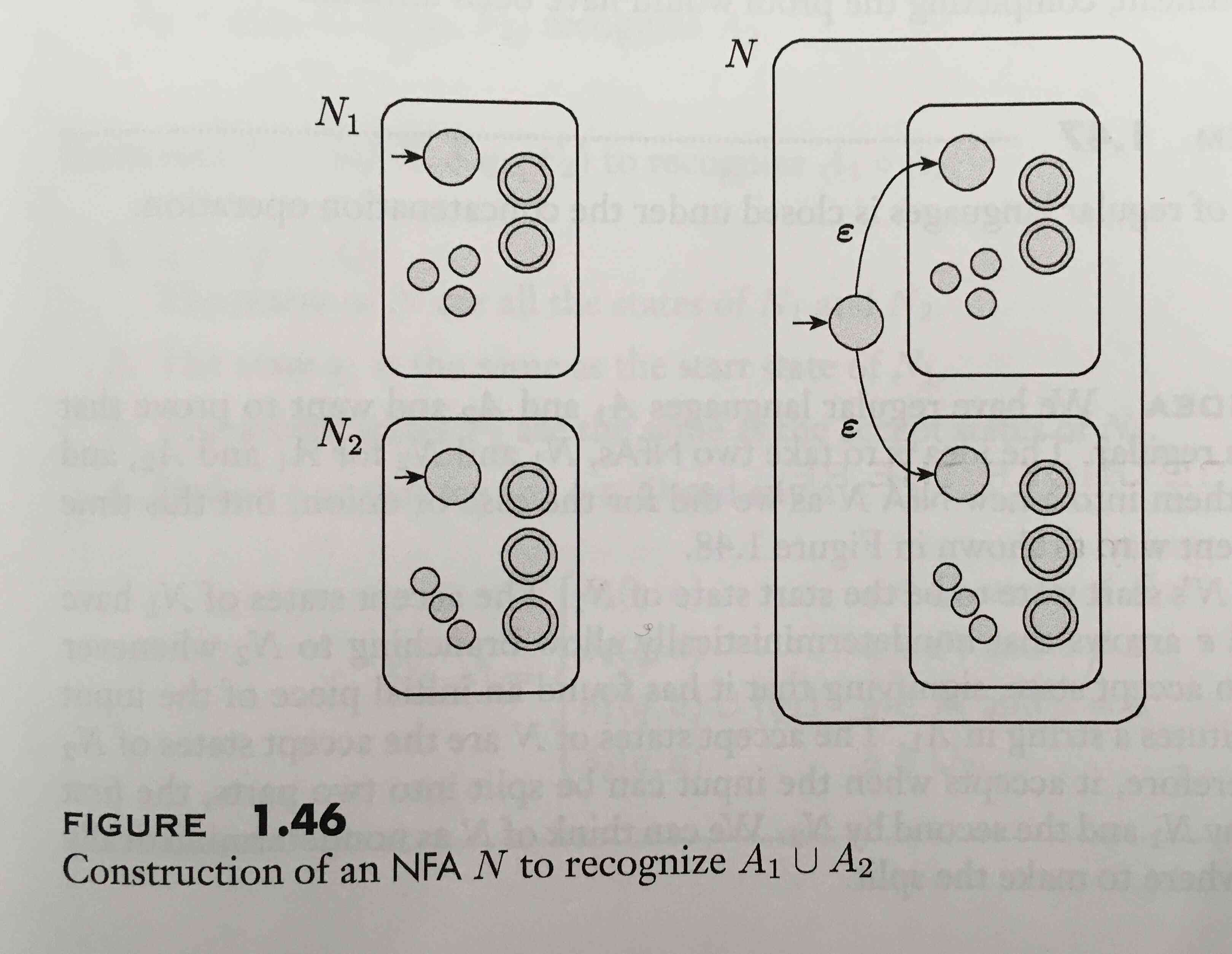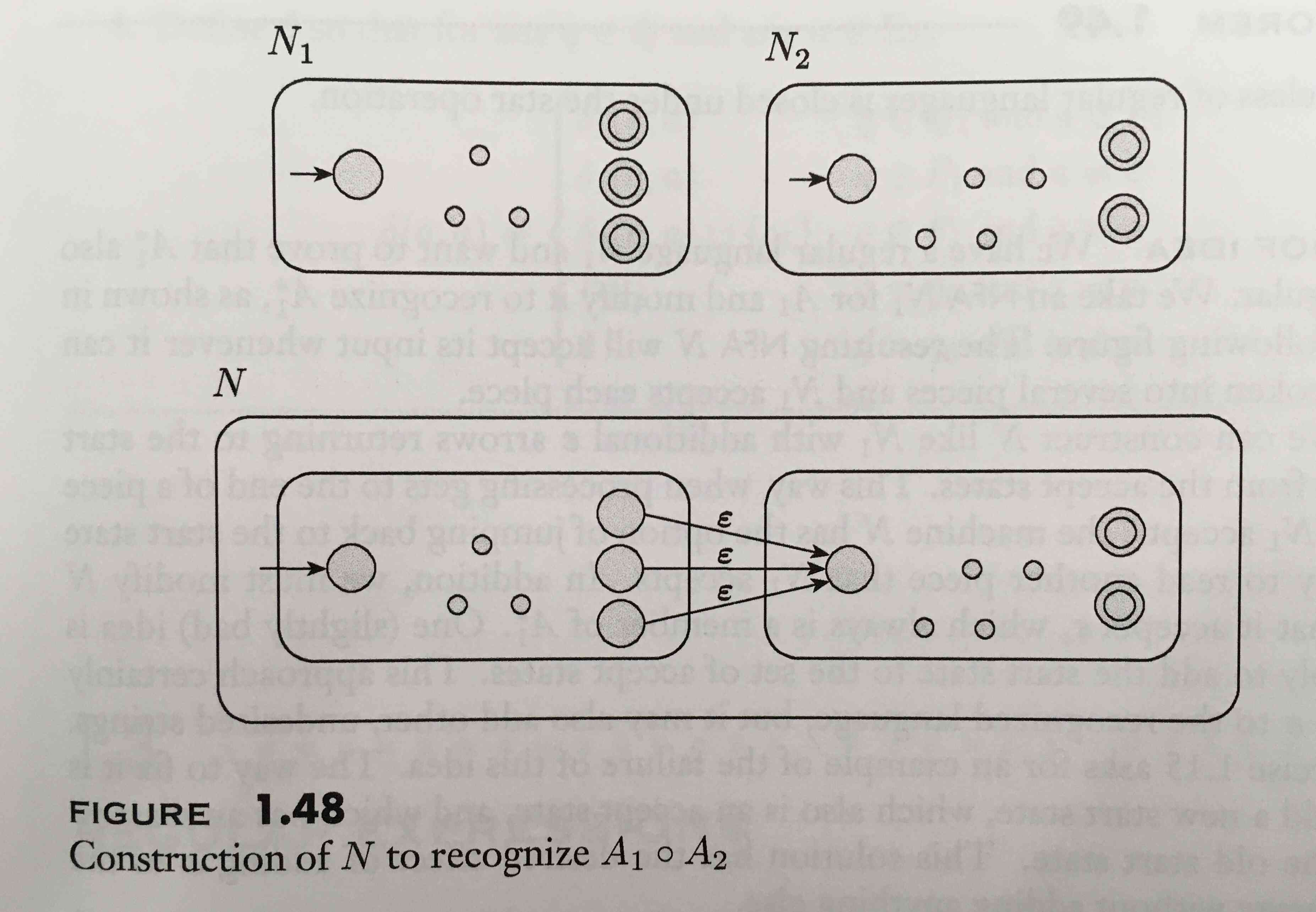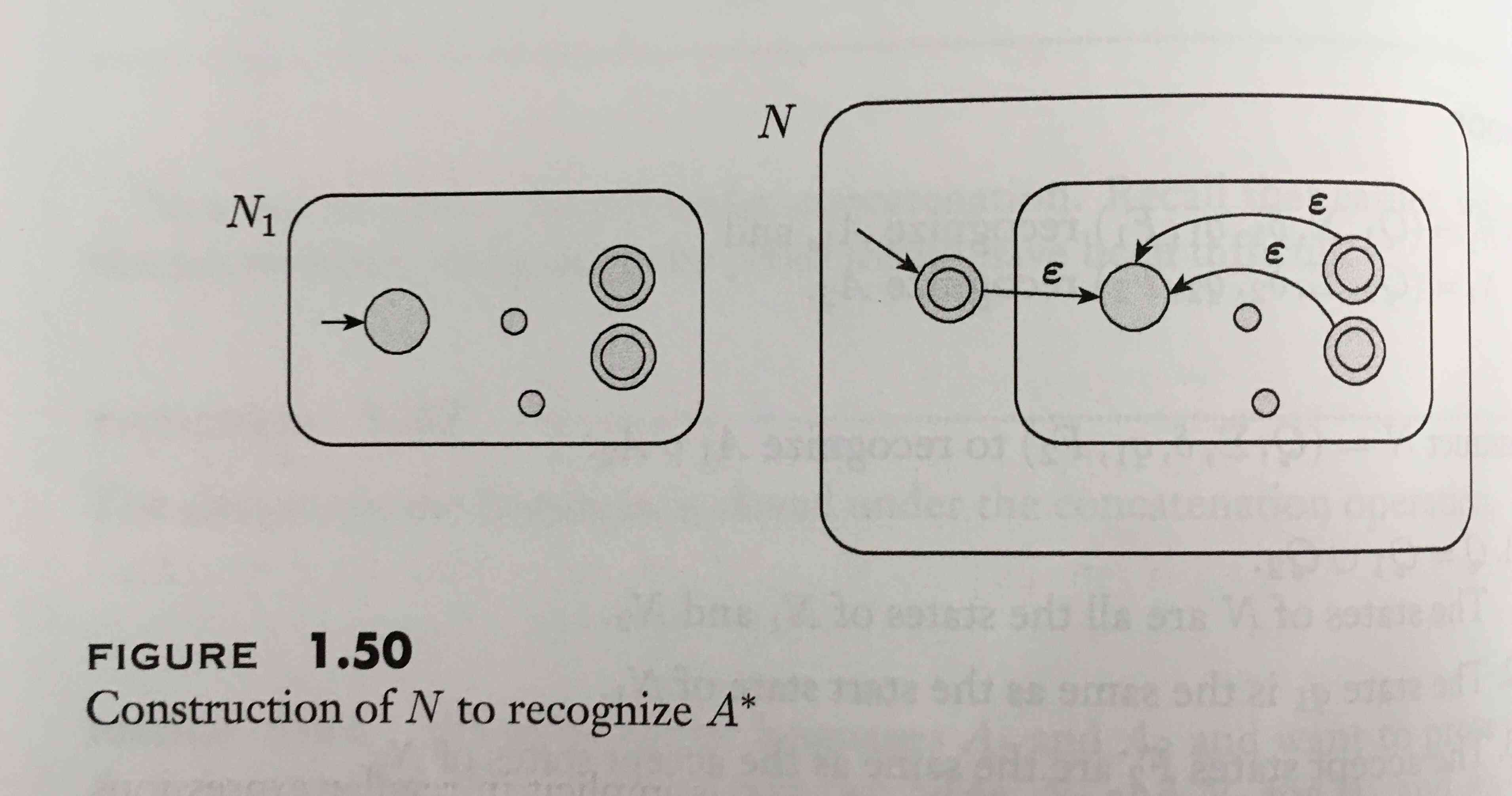Show Question
Math and science::Theory of Computation

# Regular language operators

Let $$A$$ and $$B$$ be languages. We define the regular operations union, concatenation and star as follows:

Union
$$A \cup B = \{x \mid x \in A \lor x \in B\}$$  (i.e. as per standard axiom of union for sets)
Concatenation
$$A \circ B = \{ xy \mid x \in A \land y \in B \}$$
Star
$$A^\star = \{ x_1x_2...x_k \mid k \ge 0 \text{ and each } x_i \in A \}$$

The class of regular languages is closed under these three operations!

### Visual Justification

It is quite easy to grasp how regular languages are closed under the above three operations. To do so, consider the state diagrams representing two nondeterministic finite automata $$N_1$$ and $$N_2$$ which accepts languages $$A_1$$ and $$A_2$$ respectively. We can combine them in different ways to achieve union, concatenation and star operations.

#### Union, $$A_1 \cup A_2$$

The set of start states is the union of the start states of $$N_1$$ and $$N_2$$.#### Concatenation, $$A_1 \circ A_2$$

Each accept state of $$N_1$$ has an ε-transition to the start state of $$N_2$$.#### Star operator, $$A_1^{\star}$$

Each accept state of $$N_1$$ has an ε-transition to the start state of $$N_1$$.#### Source

Introduction to the Theory of Computation, Sipser
p44